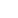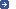– the intergovernmental organization through which Member States act together
on matters related to measurement science and measurement standards.
 Search facility:| Site map | News | Contact us> You are here: BIPM work programme > Mass > watt balance > principlesThe principle of the Kibble balanceThe concept of the moving coil watt balance was proposed by B. P. Kibble (NPL) in 1975. The experiment consists of two parts: the weighing and the moving experiments.Weighing experimentMoving experimentIn the weighing experiment, a mass and a coil are suspended from a balance. The coil (wire length L) is placed in a magnetic field of flux density B. The gravitational force on the mass m is balanced by an equal and opposite electromagnetic force on the coil by sending a current I through it: m g = I L B In the moving experiment, the coil is moved at a vertical speed v through the magnetic field so that a voltage U is induced: U = B L v

If the properties of the coil and the magnetic field, L and B, and their alignment, do not change between the two experiments, they can be eliminated from both equations, which leads to the new equation

U I = m g v

which shows an electrical power on the left-hand side and a mechanical power on the right-hand side. This explains the name of the experiment, since the watt is the unit of power. It is important to understand that both types of power are only "virtual", in the sense that they do not appear in one of the single phases of the experiment. In the case of the electrical power, the voltage is measured in the moving experiment, and the current in the weighing experiment.

To establish a link between the macroscopic mass m and the Planck constant h, the electrical quantities voltage and current are measured by using two macroscopic quantum phenomena, the Josephson effect and the quantum Hall effect.

The Josephson effect allows us to determine an unknown voltage U as a dimensionless multiple u' of a combination of the Planck constant, the elementary charge and a precisely measurable frequency fJ:

 U = u' fJ h = u' fJ2e KJ

The quantum Hall effect allows us to determine an unknown resistance R as a dimensionless multiple r' of a different combination of the Planck constant and the elementary charge:

 R = r' h = r' RKe2

The application of both effects on the measurement of the voltage and the current in the Kibble balance equation leads to:

 m = u1' u2' fJ,1 fJ,2 1 hr' g v 4

where the first component on the right-hand side shows the quantities related to the measurement of the voltage and the current using the macroscopic quantum effects, the second component shows the mechanical measurands gravitational acceleration g and velocity v, and the last term is the Planck constant. This equation establishes the link between the macroscopic mass m and the Planck constant h.SummaryGeneral descriptionGravimetryMechanical systemElectrical systemMagnetic field alignmentMagnetEnvironmentOptical systemThe principle of the Kibble balanceThe BIPM Kibble (watt) balance: general conceptPresent statusBIPM - Pavillon de Breteuil F-92312 Sèvres Cedex FRANCE DisclaimerPrivacy Policy Copyright © BIPM all rights reserved